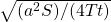# 5.4 Estimating the Magnitude and Timing of Streamflow Depletion

The factors controlling the timing of streamflow depletion by groundwater capture are essentially the same factors that control the response of an aquifer to a pumping well. These include the geometry, dimensions, and hydraulic properties of the aquifer; the locations and hydraulic characteristics of aquifer boundaries, including streams; and the horizontal and vertical distances between wells and streams (Barlow and Leake, 2012). Barlow and Leake (2012) note that the two most important factors are the distance between a pumping well and a nearby stream and the hydraulic diffusivity of the aquifer (which is defined as the ratio of the transmissivity to storage coefficient). The magnitude of the streamflow depletion will be proportional to the magnitude of the rate of pumping, although the timing is not affected by the pumping rate.

Perhaps the best way to predict the magnitude and timing of streamflow depletion in response to pumping is to develop and calibrate a reliable simulation model that accurately (or adequately) represents all of the governing factors in a stream-aquifer system. Numerical groundwater modeling software, such as the public-domain MODFLOW software, offer a framework for accomplishing this. Numerical models can readily account for heterogeneities and irregular or nonlinear boundaries. However, if certain simplifying assumptions about the system can reasonably be made, then it is possible to apply an analytical solution, which can offer a faster way to make an estimate (e.g., Glover and Balmer, 1954; Theis and Conover, 1963). Commonly required assumptions include (but are not limited to): (1) a semi-infinite, homogeneous, isotropic aquifer, (2) aquifer transmissivity remains constant with time, and (3) the bounding stream is a straight line and fully penetrates the aquifer. As summarized by Barlow and Leake (2012), Glover’s analytical solution allows one to compute the total rate of streamflow depletion with time, Qs(t), as shown in Equation 5.

 Qs(t) = Qw erfc(z) (5)

where:

 Qs(t) = total rate of streamflow depletion with time (L3/T) Qw = pumping rate of the well (L3/T) erfc = complementary error function (dimensionless) z =(dimensionless) a = distance from the well to the stream (L) S = storage coefficient (dimensionless) T = transmissivity (L2/T) t = time (T)

To simplify the mathematical complexities of the analytical solutions, Jenkins (1968) used a semi‑analytical approach in which he introduced the concept of a stream depletion factor (sdf), which had units of time and was defined as Equation 6.

 sdf = a2/D (6)

where:

 D = hydraulic diffusivity, D = T/S (L2/T)

As described by Barlow and Leake (2012), the value of sdf for a given pumping location is a relative measure of how fast streamflow depletion will occur in response to new pumping. A high value of D will result in a relatively low value of sdf and a relatively fast response of streamflow depletion to pumping. Values of sdf at every location in an aquifer can be calculated using a numerical model and then mapped for use by water managers to readily assess and compare potential impacts of new wells on streamflow for various well locations.

As an illustrative example of streamflow depletion dynamics, we can look at northeastern Arizona, USA, where base flow of streams is maintained in part by groundwater discharge from the C aquifer. Leake et al. (2005) developed a numerical model of this aquifer to help assess the possible effects of the proposed withdrawals from the C aquifer. They calculated potential streamflow depletion for two possible pumping scenarios (Figure 15). The two withdrawal scenarios were simulated for a 51‑year period of withdrawals followed by 50 years during which there were no withdrawals. Scenario A included a nearly constant withdrawal rate of about 9.0 ft3/s (0.25 m3/s), and scenario B simulated a more variable pumping rate with a maximum withdrawal rate of about 15.9 ft3/s (0.45 m3/s). The results show that streamflow depletion was undetectable for the first few years after withdrawals began, but then increased steadily during the rest of the withdrawal period. However, the depletion rate at the end of the withdrawal period was between 0.3 and 0.4 ft3/s (0.008 and 0.011 m3/s) for both scenarios ‑‑ substantially less than the withdrawal rates, largely because of the large distances of 20 miles or more between the wells and the streams.

An important lesson in these results is that the streamflow depletion continued to grow for decades after the pumping had stopped (Figure 15). These lag times complicate the management of water resources in stream-aquifer systems.Figure 15 – Streamflow depletion as a function of time for two scenarios of groundwater pumping from the C aquifer, northeastern Arizona, USA (from Barlow and Leake, 2012, as modified from Leake et al., 2005).